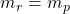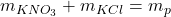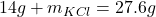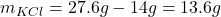Question

— A scientist combines 14 grams of potassium nitrate with an unknown amount of
potasslum chloride. If the total mass of the products is 27.6 grams, what was the
mass of the potassium chloride?

1.RuslanHeatt

The unknown amount of potassium chloride is 13.6 grams.

Explanation:

The reaction of 14 grams of KNO₃ with KCl produces a total mass of 27.6 grams of the products.

The law of conservation of mass tells us that the total mass of the reactants must be the same that the total mass of the products. So, we can find the mass of KCl as follows:Where r is for reactants and p is for productsTherefore, the unknown amount of potassium chloride is 13.6 grams.

I hope it helps you!